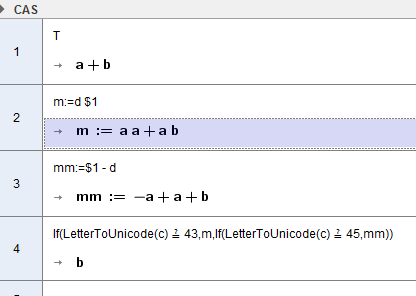# Two IF-sentences doesn’t work in CAS-view

Aritmometer shared this problem 2 years ago
Not a Problem

I wondered why I couldn’t get a IF-sentence to work in CAS-view, and found out that the following simple IF-sentence combinations doesn’t work in CAS-view.

1.

c = “+”

T = “(a + b)”

\$1 = a

\$2 = T

\$3 = If[ IsEqual[ c, “+” ] == true, \$1 * \$2, If[ IsEqual[ c, “-“ ] == true, \$2 - \$1 ] ]

This input can’t be used.

But this work:

\$3 = \$1 * \$2

\$3 = \$2 - \$1

2.

d = “a”

c = “+”

T = “(a + b)”

\$1 = T

\$2 = If[ IsEqual[ c, “+” ] == true, d * \$1, If[ IsEqual[ c, “-“ ] == true, \$1 - d ] ]

This input can’t be used.

But this work:

\$2 = d * \$1

\$2 = \$1 - d

I need to use those kinds of IF-sentences in CAS-view when the students use a dropdown-menu to choose the kind of operation they would like to use to isolate a letter in a formula and also get a description shown in text that describes what have been done and to get the formulas written in a table where they are aligned by their equal sign.1

2) IsEqual() isn't a CAS command so there's no point using it there instead of the Algebra View - you can use == instead

3) There's no point using the CAS for checking Strings anyway, just make sure it has a label and you can do

```c=="+"
```
or (more robust)

```c+"" == "+"
```

in the Algebra View1

Yes, I tried your suggestions first. But it didn’t work in CAS-view as long as one of the sides was a text-string with “” around it.

I then found out that if I used the command IsEqual[] in CAS-view, then it worked with the text-string with “” around it.1

The IF-sentence was placed in CAS-view from a click-script.1

I have been doing some tests